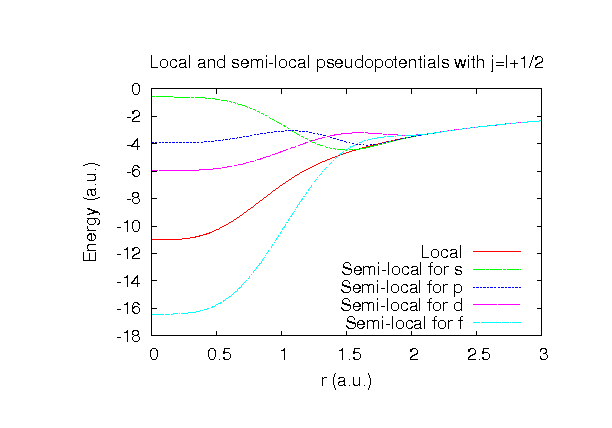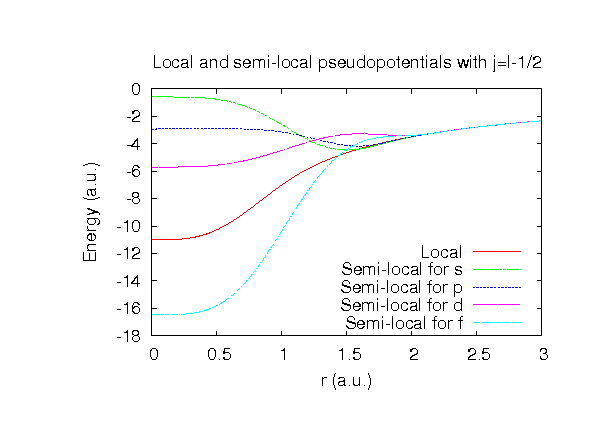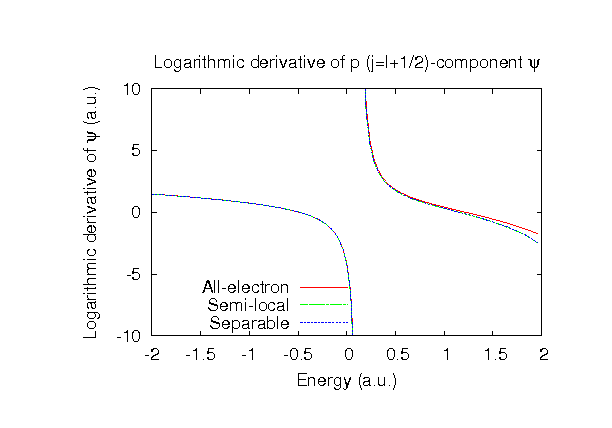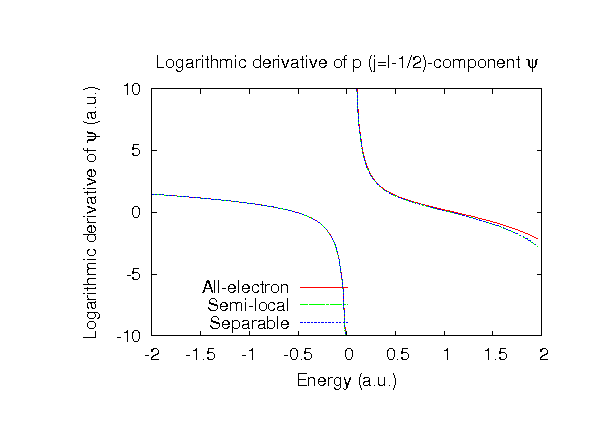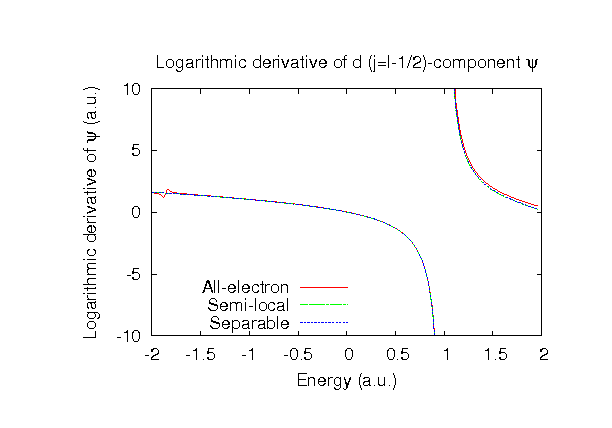### Fully relativistic pseudopotentials

Fully relativistic pseudopotentials generated by the MBK (PRB 47, 6728 (1993)) scheme within LDA (CA13) and GGA (PBE13) which contain a partial core correction and fully relativistic effects including spin-orbit coupling.

### Pseudo-atomic orbitals

The number below the symbol means a cutoff radius (a.u.) of the confinement potential. These file includes fifteen radial parts for each angular momentum quantum number l (=0,1,2,3). The basis functions were generated by variationally optimizing the corresponding primitive basis functions in the single atom, the dimer molecule, and the NaI bulk. The input files used for the orbital optimization can be found at I_opt.dat and NaI_opt.dat. Since I_CA13.vps and I_PBE13.vps include the 5s and 5p (7 electrons) as the valence states, the minimal basis set is I*.*-s1p1. Our recommendation for the choice of cutoff radius of basis functions is that I7.0.pao is enough for bulks, but I9.0.pao or I11.0.pao is preferable for molecular systems.

### Benchmark calculations by the PBE13 pseudopotential with the various basis functions

(1) Calculations of the total energy of NaI in the rock salt structure as a function of lattice constant, where the total energy is plotted relative to the minimum energy for each case. a0 and B0 are the equilibrium lattice constant and bulk modulus obtained by fitting to the Murnaghan equation of state. The difference between I7.0-s3p3d2f1 and I7.0-s3p3d3f2 in the total energy at the minimum point is 0.0032 eV/atom, where Na9.0-s3p3d2 was used as basis functions for Na for both the cases. An input file used for the OpenMX calculations can be found at NaI-EvsV.dat. For comparison the result by the Wien2k code is also shown, where the calculation was performed by default setting in the Ver. 9.1 of Wien2k except for the use of RMT x KMAX of 12.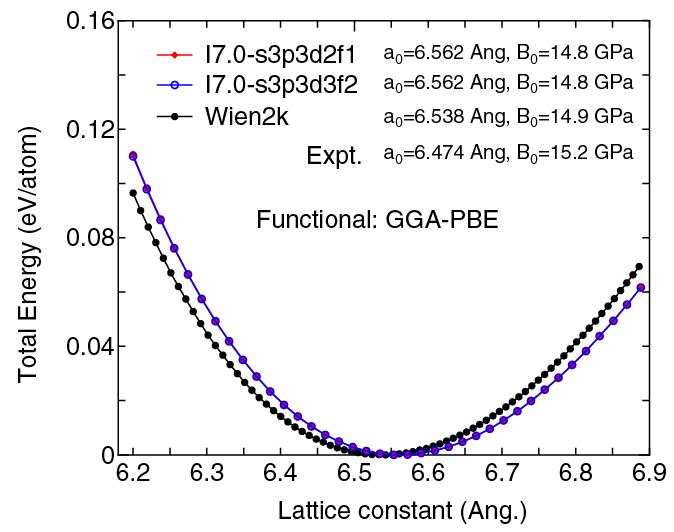(2) Calculations of the band dispersion of NaI in the rock salt structure, where the non-spin polarized collinear calculation with the lattice constant of 6.473 Ang. was performed using Na_PBE.vps, I_PBE13.vps, Na9.0-s3p3d2, and I7.0-s3p3d2f1, and the origin of the energy is taken to be the top of the valence band. The input file used for the OpenMX calculations can be found at NaI-Band.dat. For comparison the result by the Wien2k code is also shown, where the calculation was performed by default setting in the Ver. 9.1 of Wien2k except for the use of RMT x KMAX of 12.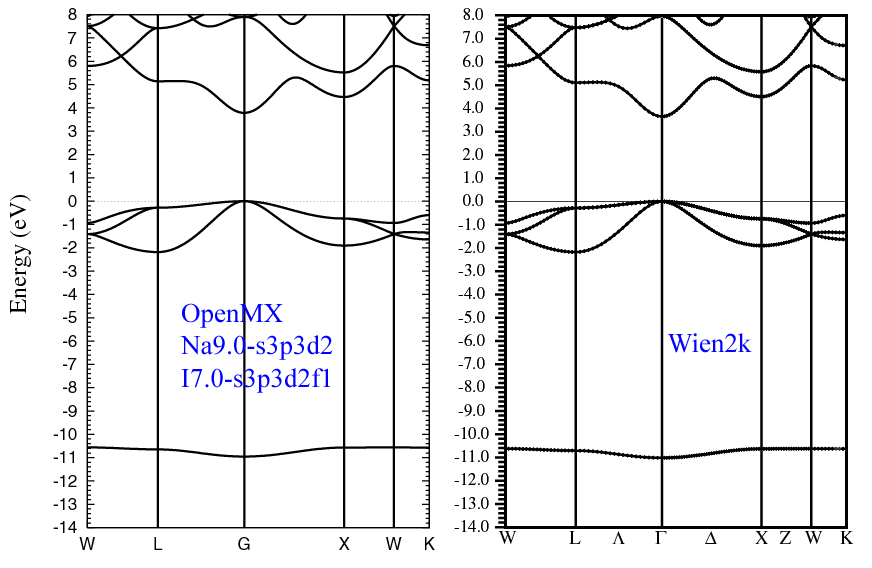### Supplementary information for the GGA (PBE13) pseudopotential